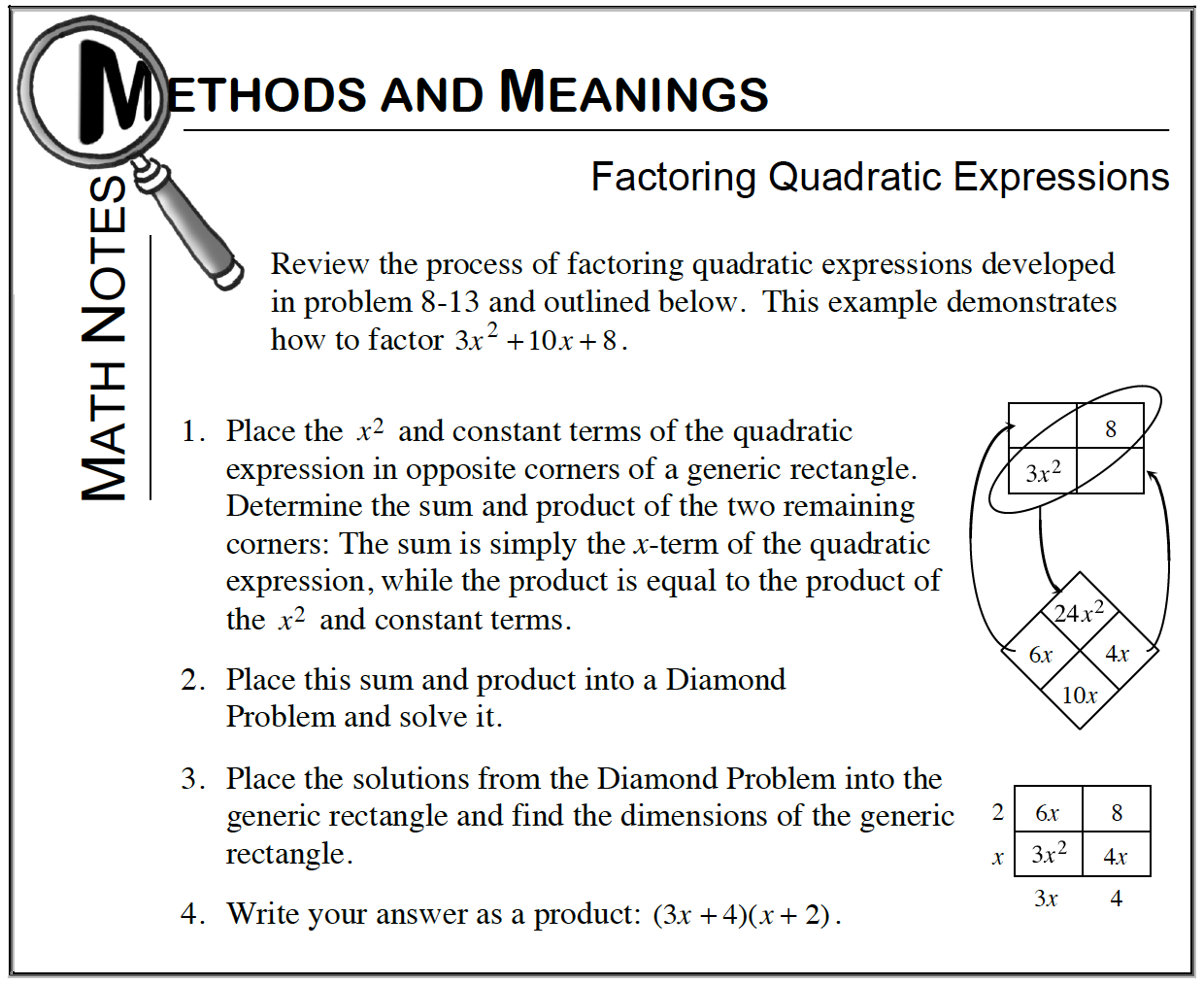Home > CCA > Chapter 9 > Lesson 9.1.3 > Problem9-33

9-33.

Factor each polynomial completely. Homework Help ✎

1. $3x^3−3x^2$

Factor out $3x^2$ from each term in the expression.

1. $2x^2−10x+12$

Begin by factoring out a $2$ from each term in the expression.

1. $8x^2−32$

After factoring out an $8$ from each term in the expression, this polynomial is the difference of two squares.

1. $4x^3+10x^2−24x$

Begin by factoring out a $2x$ from each term in the expression.

$2x(2x−3)(x+4)$